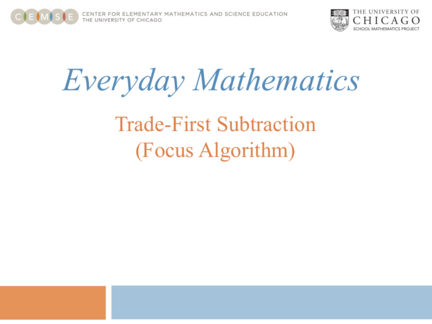Contributed by:• Figuring out the place value of digits,
• Trading first before doing any subtraction, and
• Focusing on the subtraction in one place-value column at a time
1. Everyday Mathematics
Left-to-Right Subtraction
(Focus Algorithm)Algorithm
• Figuring out the place value of digits,
• Trading first before doing any subtraction, and
• Focusing on the subtraction in one place-value column at a time.
Everyday Mathematics
method of subtracting. The key difference is that all trades are completed
before any subtracting.
You can perform the trades beginning from the right or the left. When first
introduced to the algorithm, children may prefer to draw vertical lines
separating the columns.
Solve 9,231 – 4,557 using the trade-first subtraction
Everyday Mathematics
Solve 9,231 – 4,557.
Set up the problem.
Write the numbers in their
place-value columns. 9, 2 3 1
− 4, 5 5 7
Everyday Mathematics
Solve 9,231 – 4,557.
8 12
9, 2 3 1
Go to the thousands place and
break one of the 9 [1000s] − 4, 5 5 7
into 10 [100s]. We now have
8 [1000s] and 12 [100s].
Everyday Mathematics
Solve 9,231 – 4,557.
11
8 12 13
Go to the hundreds place and 9, 2 3 1
break one of the 12 [100s]
into 10 [10s]. We now have − 4, 5 5 7
11 [100s] and 13 [10s].
Everyday Mathematics
Solve 9,231 – 4,557.
11 12
8 12 13 11
Go to the tens place and 9, 2 3 1
break one of the 13 [10s] −
into 10 [1s]. We now have 4, 5 5 7
12 [10s] and 11 [1s].
Everyday Mathematics
Solve 9,231 – 4,557
At this point, the order in 11 12
which the steps are completed 8 12 13 11
will not matter.
9, 2 3 1
Subtract in the thousands, the
hundreds, the tens, and the − 4, 5 5 7
ones column. 4, 6 7 4
Everyday Mathematics
9,231 – 4,557 = 4,674
Note that when children use the trade-first subtraction algorithm to solve an
subtraction problem, they have an opportunity to practice a variety of skills
related to developing number sense and algebraic reasoning.
These skills include:
• Writing numbers according to the place value of the digits,
• Breaking a hundred as 10 [10s]; breaking a ten as 10 [1s], and
• Renaming numbers after shifting a group of 10 [10s] or a group of 10 [1s]
to the column on its right.
Everyday Mathematics